# Perron integral

A generalization of the concept of the Lebesgue integral. A functionis said to be integrable in the sense of Perron overif there exist functions(a major function) and(a minor function) such that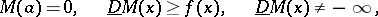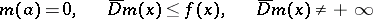(and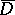are the upper and lower derivatives) for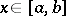, and if the lower bound to the values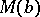of the majorantsis equal to the upper bound of the values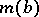of the minorants. Their common value is called the Perron integral ofoverand is denoted by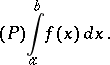The Perron integral recovers a function from its pointwise finite derivative; it is equivalent to the narrow Denjoy integral. The Perron integral for bounded functions was introduced by O. Perron , while the final definition was given by H. Bauer .

How to Cite This Entry:
Perron integral. T.P. Lukashenko (originator), Encyclopedia of Mathematics. URL: http://encyclopediaofmath.org/index.php?title=Perron_integral&oldid=11984
This text originally appeared in Encyclopedia of Mathematics - ISBN 1402006098